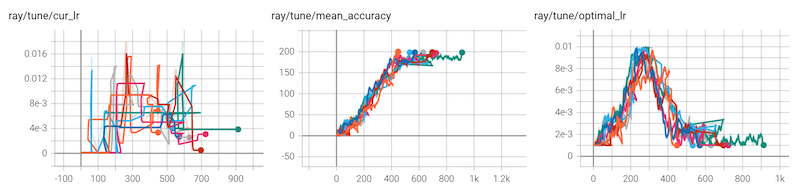# PBT Function Example#

The following script produces the following results. For a population of 8 trials, the PBT learning rate schedule roughly matches the optimal learning rate schedule.```#!/usr/bin/env python

import numpy as np
import argparse
import random

import ray
from ray import air, tune
from ray.air import session
from ray.air.checkpoint import Checkpoint
from ray.tune.schedulers import PopulationBasedTraining

def pbt_function(config):
"""Toy PBT problem for benchmarking adaptive learning rate.

The goal is to optimize this trainable's accuracy. The accuracy increases
fastest at the optimal lr, which is a function of the current accuracy.

The optimal lr schedule for this problem is the triangle wave as follows.
Note that many lr schedules for real models also follow this shape:

best lr
^
|    /\
|   /  \
|  /    \
| /      \
------------> accuracy

In this problem, using PBT with a population of 2-4 is sufficient to
roughly approximate this lr schedule. Higher population sizes will yield
faster convergence. Training will not converge without PBT.
"""
lr = config["lr"]
checkpoint_interval = config.get("checkpoint_interval", 1)

accuracy = 0.0  # end = 1000

# NOTE: See below why step is initialized to 1
step = 1
if session.get_checkpoint():
state = session.get_checkpoint().to_dict()
accuracy = state["acc"]
last_step = state["step"]
# Current step should be 1 more than the last checkpoint step
step = last_step + 1

# triangle wave:
#  - start at 0.001 @ t=0,
#  - peak at 0.01 @ t=midpoint,
#  - end at 0.001 @ t=midpoint * 2,
midpoint = 100  # lr starts decreasing after acc > midpoint
q_tolerance = 3  # penalize exceeding lr by more than this multiple
noise_level = 2  # add gaussian noise to the acc increase

# Let `stop={"done": True}` in the configs below handle trial stopping
while True:
if accuracy < midpoint:
optimal_lr = 0.01 * accuracy / midpoint
else:
optimal_lr = 0.01 - 0.01 * (accuracy - midpoint) / midpoint
optimal_lr = min(0.01, max(0.001, optimal_lr))

# compute accuracy increase
q_err = max(lr, optimal_lr) / min(lr, optimal_lr)
if q_err < q_tolerance:
accuracy += (1.0 / q_err) * random.random()
elif lr > optimal_lr:
accuracy -= (q_err - q_tolerance) * random.random()
accuracy += noise_level * np.random.normal()
accuracy = max(0, accuracy)

checkpoint = None
if step % checkpoint_interval == 0:
# Checkpoint every `checkpoint_interval` steps
# NOTE: if we initialized `step=0` above, our checkpointing and perturbing
# would be out of sync by 1 step.
# Ex: if `checkpoint_interval` = `perturbation_interval` = 3
# step:                0 (checkpoint)  1     2            3 (checkpoint)
# training_iteration:  1               2     3 (perturb)  4
checkpoint = Checkpoint.from_dict({"acc": accuracy, "step": step})

session.report(
{
"mean_accuracy": accuracy,
"cur_lr": lr,
"optimal_lr": optimal_lr,  # for debugging
"q_err": q_err,  # for debugging
"done": accuracy > midpoint * 2,  # this stops the training process
},
checkpoint=checkpoint,
)
step += 1

def run_tune_pbt(smoke_test=False):
perturbation_interval = 5
pbt = PopulationBasedTraining(
time_attr="training_iteration",
perturbation_interval=perturbation_interval,
hyperparam_mutations={
# distribution for resampling
"lr": tune.uniform(0.0001, 0.02),
# allow perturbations within this set of categorical values
"some_other_factor": [1, 2],
},
)

tuner = tune.Tuner(
pbt_function,
run_config=air.RunConfig(
name="pbt_function_api_example",
verbose=False,
stop={
# Stop when done = True or at some # of train steps
# (whichever comes first)
"done": True,
"training_iteration": 10 if smoke_test else 1000,
},
failure_config=air.FailureConfig(
fail_fast=True,
),
checkpoint_config=air.CheckpointConfig(
checkpoint_score_attribute="mean_accuracy",
num_to_keep=2,
),
),
tune_config=tune.TuneConfig(
scheduler=pbt,
metric="mean_accuracy",
mode="max",
num_samples=8,
),
param_space={
"lr": 0.0001,
# Note: `some_other_factor` is perturbed because it is specified under
# the PBT scheduler's `hyperparam_mutations` argument, but has no effect on
# the model training in this example
"some_other_factor": 1,
# Note: `checkpoint_interval` will not be perturbed (since it's not
# included above), and it will be used to determine how many steps to take
# between each checkpoint.
# We recommend matching `perturbation_interval` and `checkpoint_interval`
# (e.g. checkpoint every 4 steps, and perturb on those same steps)
# or making `perturbation_interval` a multiple of `checkpoint_interval`
# (e.g. checkpoint every 2 steps, and perturb every 4 steps).
# This is to ensure that the lastest checkpoints are being used by PBT
# when trials decide to exploit. If checkpointing and perturbing are not
# aligned, then PBT may use a stale checkpoint to resume from.
"checkpoint_interval": perturbation_interval,
},
)
results = tuner.fit()

print("Best hyperparameters found were: ", results.get_best_result().config)

if __name__ == "__main__":
parser = argparse.ArgumentParser()
"--smoke-test",
action="store_true",
default=False,
help="Finish quickly for testing",
)
type=str,
default=None,
required=False,
help="The address of server to connect to if using Ray Client.",
)
args, _ = parser.parse_known_args()
if args.smoke_test:
ray.init(num_cpus=2)  # force pausing to happen for test
else: